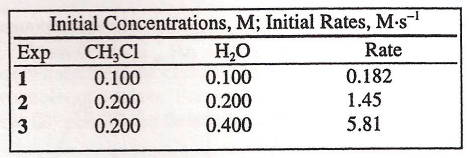# Problem: When the reaction CH3Cl(g) + H2O(g) → CH3OH(g) + HCl(g) was studied, the tabulated data were obtained. Based on these data, what are the reaction orders? (A) CH3Cl: first order         H 2O: first order (B) CH3Cl: first order         H 2O: second order (C) CH3Cl: second order     H 2O: first order (D) CH3Cl: second order     H 2O: second order

###### FREE Expert Solution
92% (336 ratings)
###### Problem Details

When the reaction CH3Cl(g) + H2O(g) → CH3OH(g) + HCl(g) was studied, the tabulated data were obtained. Based on these data, what are the reaction orders?

(A) CH3Cl: first order         H 2O: first order

(B) CH3Cl: first order         H 2O: second order

(C) CH3Cl: second order     H 2O: first order

(D) CH3Cl: second order     H 2O: second orderWhat scientific concept do you need to know in order to solve this problem?

Our tutors have indicated that to solve this problem you will need to apply the Rate Law concept. You can view video lessons to learn Rate Law. Or if you need more Rate Law practice, you can also practice Rate Law practice problems.

What is the difficulty of this problem?

Our tutors rated the difficulty ofWhen the reaction CH3Cl(g) + H2O(g) → CH3OH(g) + HCl(g) was ...as medium difficulty.

How long does this problem take to solve?

Our expert Chemistry tutor, Sabrina took 8 minutes and 59 seconds to solve this problem. You can follow their steps in the video explanation above.

What professor is this problem relevant for?

Based on our data, we think this problem is relevant for Professor Nobile's class at Marquette University.